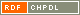# Show document

Title: On the Terwilliger algebra of bipartite distance-regular graphs with [Delta][sub]2 = 0 and c[sub]2=1 MacLean, Mark (Author)Miklavič, Štefko (Author)Penjić, Safet (Author)http://dx.doi.org/10.1016/j.laa.2016.01.040 English Not categorized 1.01 - Original Scientific Article IAM - Andrej Marušič Institute Let ▫$\Gamma$▫ denote a bipartite distance-regular graph with diameter ▫$D \geq 4$▫ and valency ▫$k \geq 3$▫. Let ▫$X$▫ denote the vertex set of ▫$\Gamma$▫, and let ▫$A$▫ denote the adjacency matrix of ▫$\Gamma$▫. For ▫$x \in X$▫ and for ▫$0 \leq i \leq D$▫, let ▫$\operatorname{\Gamma}_i(x)$▫ denote the set of vertices in ▫$X$▫ that are distance ▫$i$▫ from vertex ▫$x$▫. Define a parameter ▫$\operatorname{\Delta}_2$▫ in terms of the intersection numbers by ▫$\operatorname{\Delta}_2 = (k - 2)(c_3 - 1) -(c_2 - 1) p_{22}^2$▫. We first show that ▫$\operatorname{\Delta}_2 = 0$▫ implies that ▫$D \leq 5$▫ or ▫$c_2 \in \{1, 2 \}$▫. For ▫$x \in X$▫ let ▫$T = T(x)$▫ denote the subalgebra of ▫$\text{Mat}_X(\mathbb{C})$▫ generated by ▫$A, E_0^\ast, E_1^\ast, \ldots, E_D^\ast$▫, where for ▫$0 \leq i \leq D$, $E_i^\ast$▫ represents the projection onto the▫ $i$▫th subconstituent of ▫$\Gamma$▫ with respect to ▫$x$▫. We refer to ▫$T$▫ as the Terwilliger algebra of ▫$\Gamma$▫ with respect to ▫$x$▫. By the endpoint of an irreducible ▫$T$▫-module ▫$W$▫ we mean ▫$\min \{i | E_i^\ast W \ne 0 \}$▫. In this paper we assume ▫$\Gamma$▫ has the property that for ▫$2 \leq i \leq D - 1$▫, there exist complex scalars ▫$\alpha_i$▫, ▫$\beta_i$▫ such that for all ▫$x, y, z \in X$▫ with ▫$\partial(x, y) = 2$▫, ▫$\partial(x, z) = i$▫, ▫$\partial(y, z) = i$▫, we have ▫$\alpha_i + \beta_i | \operatorname{\Gamma}_1(x) \cap \operatorname{\Gamma}_1(y) \cap \operatorname{\Gamma}_{i - 1}(z) | = | \operatorname{\Gamma}_{i - 1}(x) \cap \operatorname{\Gamma}_{i - 1}(y) \cap \operatorname{\Gamma}_1(z) |$▫. We additionally assume that▫ $\operatorname{\Delta}_2 = 0$▫ with ▫$c_2 = 1$▫. Under the above assumptions we study the algebra ▫$T$▫. We show that if ▫$\Gamma$▫ is not almost 2-homogeneous, then up to isomorphism there exists exactly one irreducible ▫$T$▫-module with endpoint 2. We give an orthogonal basis for this ▫$T$▫-module, and we give the action of ▫$A$▫ on this basis. distance-regular graphs, terwilliger algebra, subconstituent algebra 2016 str. 307-330 Vol. 496 0024-3795 519.17 153816339610.1016/j.laa.2016.01.040597 44Document is not linked to any category.

Average score: (0 votes) Voting is allowed only to logged in users.Hover the mouse pointer over a document title to show the abstract or click on the title to get all document metadata.